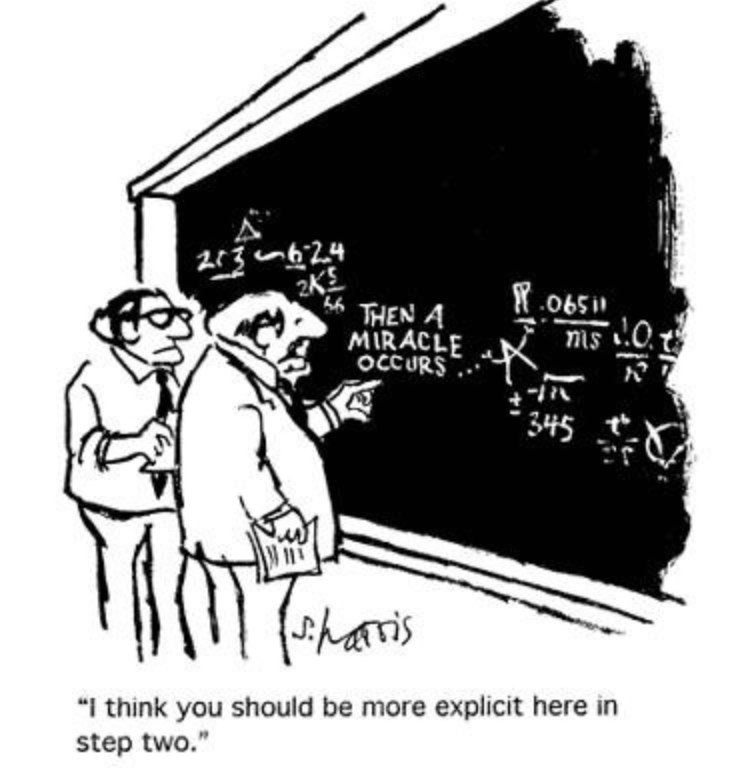# Abstracts

### Activity IHost: Michael Urbanski (UConn)

### Proof in Mathematics Education: A look into Beliefs and Conceptions

Abstract: In mathematics education, proof plays a critical role across all levels of education. The NCTM principles and Standards for School Mathematics states, "proof and proving are major aspects of school mathematics that are crucial for students to learn". Similar sentiments are echoed by the committee of the undergraduate program of mathematics. While there is an abundance of literature on students' abilities to read and write proofs for correctness, there is a growing body of work that looks into their epistemological view of proof. In this activity, we will have an active discussion about the nature, role, and acceptance of proof.

### Orthogonal Polynomials: when Analysis Meets Linear Algebra

Abstract: Orthogonal polynomials have numerous applications in probability, combinatorics, numerical analysis, physics and more. In this activity we introduce orthogonal polynomials and share some of their properties. We will then have an active discussion that incorporates calculus and linear algebra to prove a couple of interesting facts about zeros of orthogonal polynomials.

### Activity IIHost: Rachel Bailey (UConn)

### Activity III

Host: Liangbing Luo (UConn)

### Celebrating the AWM (Association for Women in Mathematics) 50th Anniversary

Abstract: First, we will give a brief history of AWM and introduce several notable female mathematicians. Then, we will present the AWM Student Chapter at UConn and some activities held by it.

### An Introduction to Rootfinding and Approximation Theory

Abstract: In this talk we give an introduction to Approximation Theory, with a focus on numerical methods used for finding the roots of functions. After reviewing both Lagrange and Chebyshev Interpolants, we explore both their theory and application in rootfinding for nonlinear problems. Finally, we provide some truly remarkable implementations of rootfinding in MATLAB applied to the gamma function as well as the Gauss map.

### Activity IVHost: Erik Wendt (UConn)

### Poster Abstracts:

 Decategorification of HFK_n(L)  by Larry Gu (USC) Using a definition of Euler characteristic for fractionally-graded complexes based on roots of unity, we show that the Euler characteristics of Dowlin’s “sl(n)-like” Heegaard Floer knot invariants HFK_n recover both Alexander polynomial evaluations and sl(n) polynomial evaluations at certain roots of unity for links in S^3. We show that the equality of these evaluations can be viewed as the decategorified content of the conjectured spectral sequences relating sl(n) homology and HFK_n. This is joint work with Professor Andy Manion. Tensor-Based Approaches to fMRI Classification by Katherine Keegan (Mary Baldwin University) To analyze the abundance of multidimensional data, tensor-based frameworks have been developed. Traditional matrix-based frameworks extract the most relevant features of vectorized data using the matrix-SVD. However, we may lose crucial high-dimensional relationships in this process. To facilitate efficient multidimensional feature extraction, we propose a projection-based classification algorithm using the t-SVDM, a tensor-based extension of the matrix-SVD. We apply our algorithm to the StarPlus functional Magnetic Resonance Imaging (fMRI) dataset. Through our numerical experiments, we conclude that there exists a more accurate tensor-based approach to fMRI classification than the best possible equivalent matrix-based approach. Our research showcases the potential of tensor-based classification frameworks, and justifies further research into the usage of tensors for numerous other classification tasks. Viscous Relativistic Hydrodynamics by Jordan Marajh (Queen Mary University of London) What would happen if you threw honey really hard at a black hole as opposed to water? Mathematicians and physicists of the field studying Einstein's Field Equations in hopes of explaining physical phenomena know so much about ideal fluids. However, the community requires research into viscous fluids of different types, i.e. admitting different types of viscosity. We encounter those of bulk and shear viscosity in the hopes of either expanding the viscous terms of the stress-energy tensor and/or changing the physical background. Analysing the dynamical systems is a large focus point for the qualitative understanding of the fluids. Relativistic Viscous Hydrodynamics by Denis George Mih (Queen Mary University of London) We analyse the dynamics of the Friedmann-Robertson-Walker and Bianchi I cosmologies when considering a viscous fluid. By examining the role of the regulator introduced by Lichnerowicz to avoid the causality issue in Eckart theory, we formulate a dynamical system which we solve analytically in both cosmological models. Lyapunov-type inequalities for third order nonlinear equations by Brian Behrens (UConn) We derive Lyapunov-type inequalities for general third-order nonlinear equations involving multiple ψ-Laplacian operators of the form $$(\psi_{2}((\psi_{1}(u'))'))' + q(x)f(u) = 0,$$ where $$\psi_{2}\text{ and }\psi_{1}$$ are odd, increasing functions, ψ1 is sub-multiplicative and 1/ψ1 is convex, and f is a continuous function which satisfies a sign condition. Our results utilize q+ and q-, as opposed to |q| which appears in most results in the literature. Additionally, these new inequalities cover previously obtained results and notably do not require the use of Holder's or the Cauchy-Schwartz inequality, unlike other proofs. Furthermore, using the obtained inequalities, we obtain a lower bound for the number of zeroes and properties of oscillatory solutions. We conclude with a constraint on the location of the maximum of a solution. Properties of Reduced Convex Hulls by Ben Arora (UConn) A set S is convex if, for any two points in S, the line segment connecting them is also in S. Given a set of points P, we define their convex hull as the smallest convex set that contains P. Sometimes, we want to shrink the convex hull of a set of points in a non-uniform manner. This gives rise to the more general concept of a "reduced convex hull." Such objects originated in binary classification problems in machine learning, and have been studied further in the field of computational geometry. We will explore various properties of reduced convex hulls, and how these properties behave as the number of points in our set gets large.